Related Articles

# Inheritance in Scala

• Last Updated : 17 Jan, 2019

Inheritance is an important pillar of OOP(Object Oriented Programming). It is the mechanism in Scala by which one class is allowed to inherit the features(fields and methods) of another class.
Important terminology:

• Super Class: The class whose features are inherited is known as superclass(or a base class or a parent class).
• Sub Class: The class that inherits the other class is known as subclass(or a derived class, extended class, or child class). The subclass can add its own fields and methods in addition to the superclass fields and methods.
• Reusability: Inheritance supports the concept of “reusability”, i.e. when we want to create a new class and there is already a class that includes some of the code that we want, we can derive our new class from the existing class. By doing this, we are reusing the fields and methods of the existing class.

#### How to use inheritance in Scala

The keyword used for inheritance is extends.
Syntax:

```class parent_class_name extends child_class_name{
// Methods and fields
}```

Example:

 `// Scala program to illustrate the ``// implementation of inheritance`` ` `// Base class``class` `Geeks``1``{``    ``var` `Name``:` `String ``=` `"Ankita"``}`` ` `// Derived class``// Using extends keyword ``class` `Geeks``2` `extends` `Geeks``1``{``    ``var` `Article``_``no``:` `Int ``=` `130``     ` `    ``// Method``    ``def` `details()``    ``{``    ``println(``"Author name: "` `+Name);``    ``println(``"Total numbers of articles: "` `+Article``_``no);``    ``}``}`` ` `object` `Main ``{``     ` `    ``// Driver code``    ``def` `main(args``:` `Array[String]) ``    ``{``         ` `        ``// Creating object of derived class``        ``val` `ob ``=` `new` `Geeks``2``();``        ``ob.details();``    ``}``}`

Output:

```Author name: Ankita
Total numbers of articles: 130
```

Explanation: In the above example Geeks1 is the base class and Geeks2 is the derived class which is derived from Geeks1 using extends keyword. In the main method when we create the object of Geeks2 class, a copy of all the methods and fields of the base class acquires memory in this object. That is why by using the object of the derived class we can also access the members of the base class.

#### Type of inheritance

Below are the different types of inheritance which are supported by Scala.

• Single Inheritance: In single inheritance, derived class inherits the features of one base class. In the image below, class A serves as a base class for the derived class B.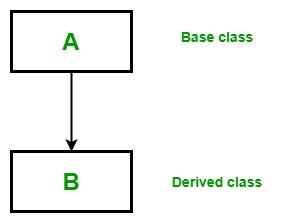Example:
 `// Scala program to illustrate the ``// Single inheritance`` ` `// Base class``class` `Parent``{``    ``var` `Name``:` `String ``=` `"Ankita"``}`` ` `// Derived class``// Using extends keyword ``class` `Child ``extends` `Parent``{``    ``var` `Age``:` `Int ``=` `22``     ` `    ``// Method``    ``def` `details()``    ``{``    ``println(``"Name: "` `+Name);``    ``println(``"Age: "` `+Age);``    ``}``}`` ` `object` `Main``{``     ` `    ``// Driver code``    ``def` `main(args``:` `Array[String]) ``    ``{``         ` `        ``// Creating object of the derived class``        ``val` `ob ``=` `new` `Child();``        ``ob.details();``    ``}``}`

Output:

```Name: Ankita
Age: 22
```
• Multilevel Inheritance: In Multilevel Inheritance, a derived class will be inheriting a base class and as well as the derived class also act as the base class to another class. In the below image, the class A serves as a base class for the derived class B, which in turn serves as a base class for the derived class C.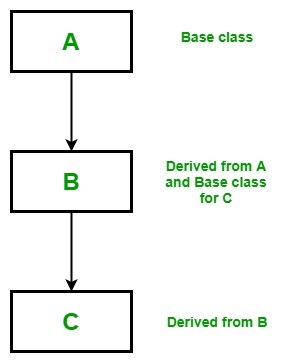Example:
 `// Scala program to illustrate the ``// Multilevel inheritance`` ` `// Base class``class` `Parent``{``    ``var` `Name``:` `String ``=` `"Soniya"``}`` ` `// Derived from parent class``// Base class for Child2 class``class` `Child``1` `extends` `Parent``{``    ``var` `Age``:` `Int ``=` `32``}`` ` `// Derived from Child1 class``class` `Child``2` `extends` `Child``1``{``    ``// Method``    ``def` `details(){``    ``println(``"Name: "` `+Name);``    ``println(``"Age: "` `+Age);``    ``}``}`` ` `object` `Main``{``     ` `    ``// Drived Code``    ``def` `main(args``:` `Array[String]) ``    ``{``         ` `        ``// Creating object of the derived class``        ``val` `ob ``=` `new` `Child``2``();``        ``ob.details();``    ``}``}`

Output:

```Name: Soniya
Age: 32
```
• Hierarchical Inheritance: In Hierarchical Inheritance, one class serves as a superclass (base class) for more than one subclass.In below image, class A serves as a base class for the derived class B, C, and D.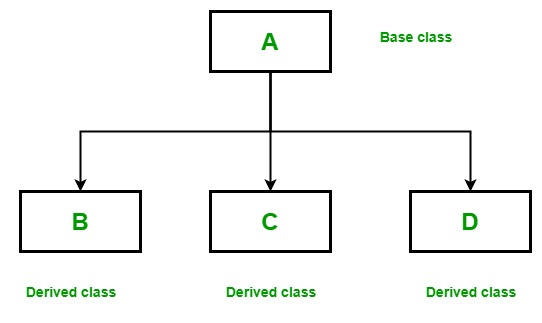Example:
 `// Scala program to illustrate the ``// Hierarchical inheritance`` ` `// Base class``class` `Parent``{``    ``var` `Name``1``:` `String ``=` `"Siya"``    ``var` `Name``2``:` `String ``=` `"Soniya"``}`` ` `// Derived from the parent class``class` `Child``1` `extends` `Parent``{``    ``var` `Age``:` `Int ``=` `32``    ``def` `details``1``()``    ``{``    ``println(``" Name: "` `+Name``1``);``    ``println(``" Age: "` `+Age);``    ``}``}`` ` `// Derived from Parent class``class` `Child``2` `extends` `Parent``{``    ``var` `Height``:` `Int ``=` `164``     ` `    ``// Method``    ``def` `details``2``()``    ``{``    ``println(``" Name: "` `+Name``2``);``    ``println(``" Height: "` `+Height);``    ``}``}`` ` `object` `Main ``{``     ` `    ``// Driver code``    ``def` `main(args``:` `Array[String]) ``    ``{``         ` `        ``// Creating objects of both derived classes``        ``val` `ob``1` `=` `new` `Child``1``();``        ``val` `ob``2` `=` `new` `Child``2``();``        ``ob``1``.details``1``();``        ``ob``2``.details``2``();``    ``}``}`

Output:

``` Name: Siya
Age: 32
Name: Soniya
Height: 164
```
• Multiple Inheritance: In Multiple inheritance ,one class can have more than one superclass and inherit features from all parent classes. Scala does not support multiple inheritance with classes, but it can be achieved by traits.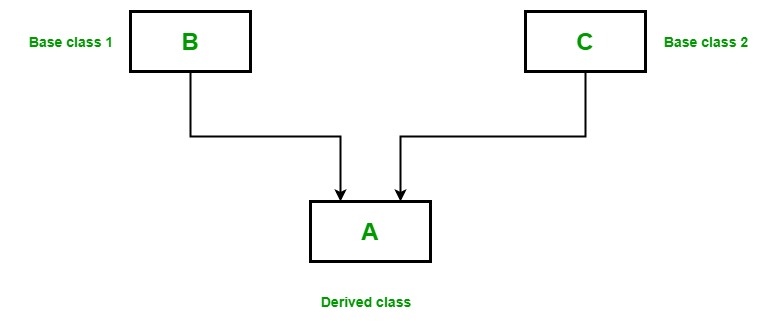Example:
 `// Scala program to illustrate the ``// multiple inheritance using traits`` ` `// Trait 1``trait` `Geeks``1``{``    ``def` `method``1``()``}`` ` `// Trait 2``trait` `Geeks``2``{``    ``def` `method``2``()``}`` ` `// Class that implement both Geeks1 and Geeks2 traits``class` `GFG ``extends` `Geeks``1` `with` `Geeks``2``{``     ` `    ``// method1 from Geeks1``    ``def` `method``1``()``    ``{``        ``println(``"Trait 1"``);``    ``}``     ` `    ``// method2 from Geeks2``    ``def` `method``2``()``    ``{``        ``println(``"Trait 2"``);``    ``}``}``object` `Main ``{``    ``// Driver code``    ``def` `main(args``:` `Array[String])``    ``{``         ` `        ``// Creating object of GFG class``        ``var` `obj ``=` `new` `GFG();``        ``obj.method``1``();``        ``obj.method``2``();``    ``}``}`

Output:

```Trait 1
Trait 2
```
• Hybrid Inheritance: It is a mix of two or more of the above types of inheritance. Since Scala doesn’t support multiple inheritance with classes, the hybrid inheritance is also not possible with classes. In Scala, we can achieve hybrid inheritance only through traits.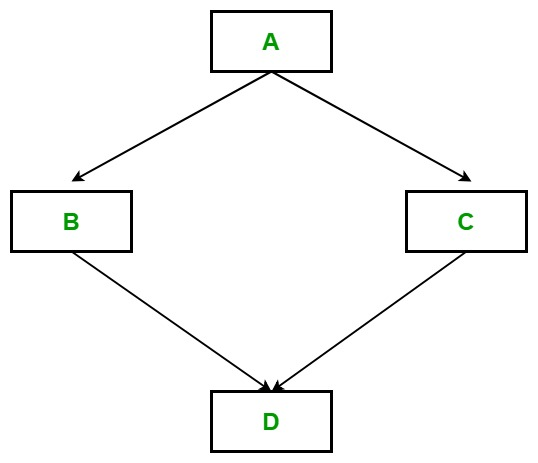My Personal Notes arrow_drop_up